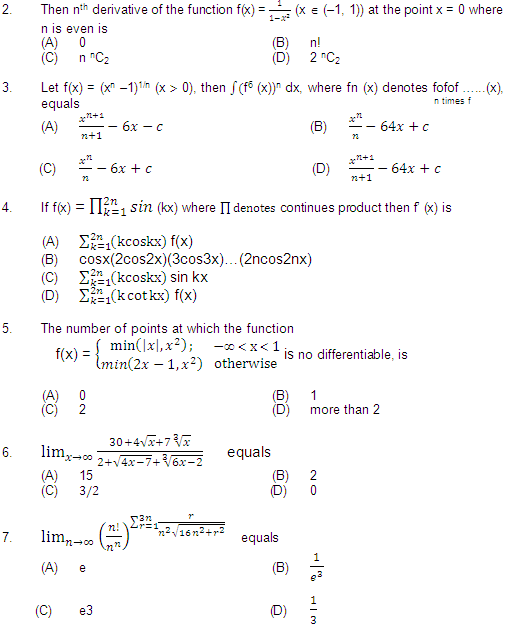Click to Chat

1800-1023-196

+91-120-4616500

CART 0

• 0

MY CART (5)

Use Coupon: CART20 and get 20% off on all online Study Material

ITEM
DETAILS
MRP
DISCOUNT
FINAL PRICE
Total Price: Rs.

There are no items in this cart.
Continue Shopping• Complete JEE Main/Advanced Course and Test Series
• OFFERED PRICE: Rs. 15,900
• View Details

```askIITians PART TEST-1

MATHEMATICS

SECTION - III

PART - A

Straight Objective Type:

This section contains 9 multiple choice questions numbered 1 to 9. Each question has 4 choices (A), (B), (C) and (D) out of which ONLY ONE is correct.

1. The order and degree of the differential equations of the family of hyperbolas centred at origin; principal axis along co-ordinate axis and of length √(a2+λ) and √(b2+λ) where a, b are fixed real numbers and λ is a real parameter is
(A) 1, 1                                                              (B) 2, 1
(C) 1, 2                                                             (D) 3, 28. Let f: [k, k + 1, …, 2007] ® [1, 2, 3, …, n] be defined by f(x) = [ 2007 / x ] (where [.] denotes the greatest integer function). The maximum value of k such that it is not possible to make f an onto function for any vale of n is
(A) 50                                                                (B) 40
(C) 51                                                               (D) none of these

9. Let f and g be continuous and differentiable functions. If f(0) = f(2) = f(4); f(1) + f(3) = 0; g(0) = g(2) = g(4) = 0 and if f(x) = 0 and g’(x) = 0 do not have a common root, then the minimum number of zeros of f’(x)g’(x) + f(x) g”(x) in [0, 4] is
(A) 3                                                                 (B) 4
(C) 5                                                                (D) 2

7

Next >>

```### Course Features

• 731 Video Lectures
• Revision Notes
• Previous Year Papers
• Mind Map
• Study Planner
• NCERT Solutions
• Discussion Forum
• Test paper with Video Solution